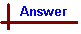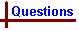REDOX EQUATIONS AND REACTIONS OF COMPLEXES (Questions) Your feedback on these self-help problems is appreciated. Click here to send an e-mail. For on-line tutorial help with redox questions, see the chemCAL module, Electrochemistry. Shortcut to Questions Q: 1 2 3 4 5 6 7 1 Write an equation for the reaction which occurs when excess 4 M ammonia solution is added to each of the following. If no reaction occurs, write "no reaction". (1.) a water solution of silver nitrate (2.) a water solution of copper(II) nitrate (3.) a water solution of zinc chloride (4.) a water solution of tetraamminecopper(II) sulfate (5.) a water solution of diammonium copper(II) sulfate2 Arsenic(III) oxide is amphoteric. Write a balanced equation for each of two reactions that establish this fact.3 What is the oxidation number of the underlined central atom in each of the following species? For on-line help with the concept of oxidation numbers, see the chemcal screen titled "Oxidation Numbers A", and in particular, the hint to that screen where the rules for deriving oxidation numbers are set out. (1.) Cs+, (2.) Bi3+, (3.) N3-, (4.) SO42-, (5.) NH3, (6.) N2H4, (7.) NO2, (8.) NO2-, (9.) PbO2, (10.) BaO, (11.) H2O2, (12.) NaH, (13.) HClO4, (14.) HClO, (15.) HBrO3, (16.) [Fe(CN)6]4-, (17.) [Cu(CN)4]3-, (18.) [SnCl6]2-, (19.) K3[Fe(CN)6], (20.) K2[PtCl6]4 Using the ion-electron half-reaction method balance the following "skeleton" redox equations: (1.) Cr2O72- + H2S(aq) → Cr3+ + S(s) (acidic solution) (2.) Cr2O72- + I- → Cr3+ + I2(s) (acidic solution) (3.) Fe3+ + I- → Fe2+ + I2(s) (4.) NO2- + MnO4- → NO3- + MnO2(s) (basic solution) (5.) Br2(l) + OH- → BrO3- + Br- (hot basic solution) (6.) H2S(aq) + NO3- → S(s) + NO2(g) (acidic solution) (7.) PbS(s) + NO3- → Pb2+ + S(s) + NO(g) (dilute acidic solution) (8.) MnO4- + Cl- → Mn2+ + Cl2(g) (acidic solution) (9.) SO2(g) + NO3- → SO42- + NO(g) (acidic solution) (10.) ClO3- → ClO2 + ClO4- (acidic solution) (11.) H2O2 + I2(s) → HIO3 (12.) S2O32- + I2(s) → S4O62- + I- (13.) Al(s) + OH- → Al(OH)4- + H2(g) (strongly basic solution) (14.) Ag(s) + H2S(g) → Ag2S(s) + H2(g) (15.) Pb(s) + PbO2(s) → Pb2+ (acidic solution)5 Using the ion-electron half-reaction method balance the following redox equations for which all reactants and products are given, and underline all reductants. (1.) H3AsO4 + Sn2+ + H+ → H3AsO3 + Sn4+ + H2O (2.) NO3- + Fe2+ + H+ → NO(g) + Fe3+ + H2O (3.) N2O4(g) + Br- + OH- → NO2- + BrO3- + H2O6 Write a balanced equation for each of the following redox reactions (1.) The oxidation of iron(II) ion to iron(III) ion by chlorine. (2.) The oxidation of nitrogen(II) oxide (NO) to nitrogen dioxide by concentrated nitric acid. (3.) The oxidation of tin(II) ion by potassium dichromate in dilute aqueous sulfuric acid solution. (4.) The reduction of iron(III) ion to iron(II) ion by hydriodic acid. (5.) The oxidation of iron(II) ion by dichromate ion in dilute sulfuric acid solution. (6.) The oxidation of hydrogen peroxide by permanganate ions in dilute sulfuric acid solution. (7.) The action of concentrated nitric acid on copper giving nitrogen dioxide. (8.) The action of excess warm dilute sodium hydroxide solution on zinc.7 (1.) Give five oxidation states of manganese. (2.) When dilute hydrochloric acid is added to a water solution of potassium manganate, the products are manganese dioxide and permanganate ion. Write an equation for this redox reaction.REDOX EQUATIONS (Answers) 1 (1.) Ag+ + NH3(aq) + H2O → AgOH(s) + NH4+, followed by: AgOH(s) + 2NH3(aq) → [Ag(NH3)2+] + OH-. This is an example of a reaction producing a product which can react further with the added ammonia (competing equilibria). In the first reaction ammonia acts as a base (ie a source of hydroxide ions), whilst in the second it acts as a ligand, coordinating to the silver atom. (2.) Cu2+ + 2NH3(aq) + 2H2O → Cu(OH)2(s) + 2NH4+, followed by: Cu(OH)2(s) + 4NH3(aq) → [Cu(NH3)42+] + 2OH- (3.) Zn2+ + 2NH3(aq) + 2H2O → Zn(OH)2(s) + 2NH4+, followed by: Zn(OH)2(s) + 4NH3(aq) → [Zn(NH3)42+] + 2OH- (4.) No reaction. Note that tetraamminecopper(II) is the ion produced in part (2.), and is the final product between copper(II) ions and NH3. (5.) Cu2+ + 2NH3(aq) + 2H2O → Cu(OH)2(s) + 2NH4+, followed by: Cu(OH)2(s) + 4NH3(aq) → [Cu(NH3)42+] + 2OH-2 (1.) As2O3(s) + 6H+ → 2As3+ + 3H2O (2.) As2O3(s) + 3H2O + 2OH- → 2[As(OH)4-] An amphoteric compound is one that can react with both H+ and OH-. In the first equation As2O3 reacts with H+ to form the arsenic(III) cation. In the second equation As2O3 reacts with OH- to form the tetrahydroxoarsenate(III) anion.3 (1.) +I, (2.) +III, (3.) -III, (4.) +VI, (5.) -III, (6.) -II, (7.) +IV, (8.) +III, (9.) +IV, (10.) +II, (11.) -I, (12.) -I, (13.) +VII, (14.) +I, (15.) +V, (16.) +II, (17.) +I, (18.) +IV, (19.) +III, (20.) +IV4 A worked solution is given for part (1.) - the other problems are approached by applying the same steps. (1.) There are five steps which provide the easiest way to balance redox equations: Step 1: Divide the unbalanced equation Cr2O72- + H2S → Cr3+ + S into two half-reactions involving the species oxidised and reduced: Cr2O72- → Cr3+ H2S → S Step 2: Balance atoms and charges in each half-reaction. The order for balancing is all atoms other than O and H, then O (by adding H2O), then H (by adding H+), and finally charges: Cr2O72- + 14H+ +6e- → 2Cr3+ + 7H2O H2S → S + 2H+ + 2e- Step 3: Multiply each half-reaction through by an integer to make electrons lost in one half-reaction equal the number gained in the other (if necessary): Cr2O72- + 14H+ +6e- → 2Cr3+ + 7H2O 3H2S → 3S + 6H+ + 6e- .... multiplied through by 3 to give 6e- lost. Step 4: Add the two half-reactions together, cancelling any common terms, and adding states of matter: Cr2O72-(aq) + 3H2S(aq) + 8H+(aq) → 2Cr3+(aq) + 3S(s) + 7H2O(l) Step 5: An extra step here to check for errors is a good idea, especially with complicated reactions - simply check that the atoms on each side actually balance. This procedure may seem long, but a systematic way to balance redox equations is essential. It is easy to see that with so many reactants, products, and coefficients, balancing a whole redox equation by trial and error would be very frustrating - without taking electron transfer into account finding the actual, correct equation is practically impossible! (2.) Cr2O72- + 6I- + 14H+ → 2Cr3+ + 3I2(s) + 7H2O (3.) 2Fe3+ + 2I- → 2Fe2+ + I2(s) (4.) 3NO2- + 2MnO4- + H2O → 3NO3- + 2MnO2(s) + 2OH- (5.) 3Br2(l) + 6OH- → BrO3- + 5Br- + 3H2O (6.) H2S(aq) + 2NO3- + 2H+ → S(s) + 2NO2(g) + 2H2O (7.) 3PbS(s) + 2NO3- + 8H+ → 3Pb2+ + 3S(s) + 2NO(g) + 4H2O (8.) 2MnO4- + 10Cl- + 16H+ → 2Mn2+ + 5Cl2(g) + 8H2O (9.) 3SO2(g) + 2NO3- + 2H2O → 3SO42- + 2NO(g) + 4H+ (10.) 3ClO3- + 2H+ → 2ClO2 + ClO4- + H2O (11.) 5H2O2 + I2(s) → 2HIO3 + 4H2O (12.) 2S2O32- + I2(s) → S4O62- + 2I- (13.) 2Al(s) + 2OH- + 6H2O → 2Al(OH)4- + 3H2(g) (14.) 2Ag(s) + H2S(g) → Ag2S(s) + H2(g) (15.) Pb(s) + PbO2(s) + 4H+ → 2Pb2+ + 2H2O5 (1.) H3AsO4 + Sn2+ + 2H+ → H3AsO3 + Sn4+ + H2O (2.) NO3- + 3Fe2+ + 4H+ → NO(g) + 3Fe3+ + 2H2O (3.) 3N2O4(g) + Br- + 6OH- → 6NO2- + BrO3- + 3H2O6 (1.) 2Fe2+ + Cl2(g) → 2Fe3+ + 2Cl- (2.) NO(g) + 2NO3- + 2H+ → 3NO2(g) + H2O (3.) 3Sn2+ + Cr2O72- + 14H+ → 3Sn4+ + 2Cr3+ + 7H2O (4.) 2Fe3+ + 2I- → 2Fe2+ + I2(s) (5.) 6Fe2+ + Cr2O72- + 14H+ → 6Fe3+ + 2Cr3+ + 7H2O (6.) 5H2O2 + 2MnO4- + 6H+ → 5O2(g) + 2Mn2+ + 8H2O Note that the above equation is the only correct one since it is derived from a consideration of electrons gained and lost. Other equations can be written, derived by trial and error - these are incorrect! (7.) Cu(s) + 2NO3- + 4H+ → Cu2+ + 2NO2(g) + 2H2O (8.) Zn(s) + 2OH- + 2H2O → Zn(OH)42- + H2(g)7 (1.) Any five of: 0, +II, +III, +IV, +VI, +VII (2.) 3MnO42- + 4H+ → 2MnO4- + MnO2(s) + 2H2O. In this reaction MnO42- acts as both oxidant and reductant.